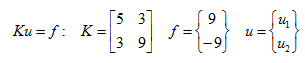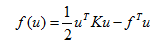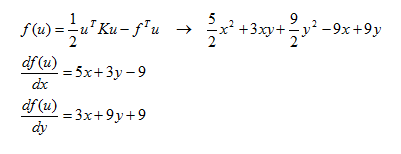you are here:/ News & Insights / Engineering Advantage Blog / Understanding the Finite Element Iterative Solver - Part I

# Understanding the Finite Element Iterative Solver - Part IFebruary 27, 2015 By: Eric Stamper

There are a couple main approaches used when solving the numerical equations in an implicit structural problem. They are commonly referred to as either a direct or iterative solving approach. The focus of this post is on iterative solving, which can be a more efficient method when solving finite element problems that contain a large number of degrees of freedom (when the problem is well posed). This method also uses less CPU memory than the direct, so it has additional advantage: a larger problem can be solved on your computing resources.

The overall goal for a static structural finite element analysis (mathematically) is to obtain the solution to a set of linear algebraic equations where there is only one unique result. This result will occur if the problem is positive definite (to be described shortly).  A popular iterative approach that is used when solving these sets of equations is the Conjugate Gradient Method combined with a Pre-conditioner. (In ANSYS this is known as the PCG solver) This method is an effective approach to iteratively solve a set of linear equations represented by: Ku=f.

Where: u is an unknown vector, f is a known vector and K is a known matrix.

It's easier to understand these concepts  by visualizing what the iterative method is trying to achieve in mathematical terms.

Think of this simple problem below.  You have a linear system of equations (two equations defined by the two lines). The solution to the system is at the intersection of these two lines:

## iterative1.pngThe system of equations representing the two lines above can be written in the following matrix form just like you would find in a finite element model:And this equation "Ku=f " is the solution to the quadratic form when it is minimized. This might be a little bit of a leap at this point, but skipping the math to demonstrate the idea behind the method, the quadratic form is:Which, for the system of equations we have, f (u) can be visualized in three dimensions with the plot below:

## iterative2.pngThe parabolic shape of the 3D curve represents a positive definite matrix, which means that it has one point at which f (u) will have a minimum value. This point can be easier to see with a contour plot in the u1-u2 plane, as shown below:

## iterative3.pngThe contour lines are closing in on the point u1=3, u2=-2 which was previously shown as the solution to the crossing lines. This is not a coincidence, since when quadratic form is minimized (e.g. the minimum point on the 3D curve is found) we have the solution. Ku= f ends up being this solution because if the derivative of this curve is obtained (e.g. the slope of the curve at any given point), the point at which the slope is zero represents the minimum value.

For the example we have, f (u) evaluates out to the following equation below, and when the two derivatives are taken, we're back to the original two equations with the solution:

f (u) = Ku- f = 0.This shows some of the basics, now the solution needs to be found in an iterative fashion. Meaning that an initial guess is made as to what the solution might be, and it's iteratively adjusted to find the minimum value on the 3D curve. Keep in mind that the conjugate gradient method works when the matrix is positive definite since its shape has one minimum point.

For an indefinite matrix, this method will not work, nor would there be one unique solution. It's easy to see that this is the case by again visualizing the shape of the quadratic form. As shown below, an indefinite matrix is saddle shaped and it's easy to identify that one unique solution does not exist.

## iterative4.pngStay tuned for part II of this post; where the minimum point (i.e. the solution!) to this problem will be found using the conjugate gradient method.